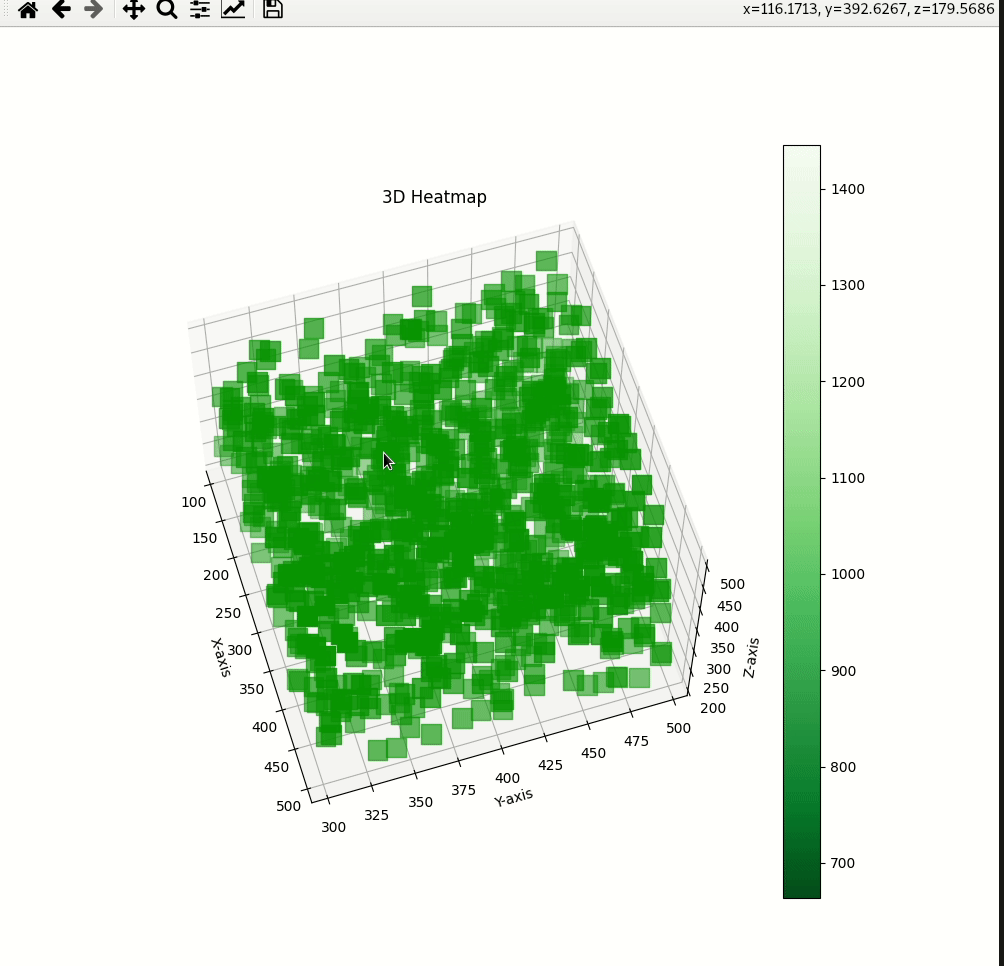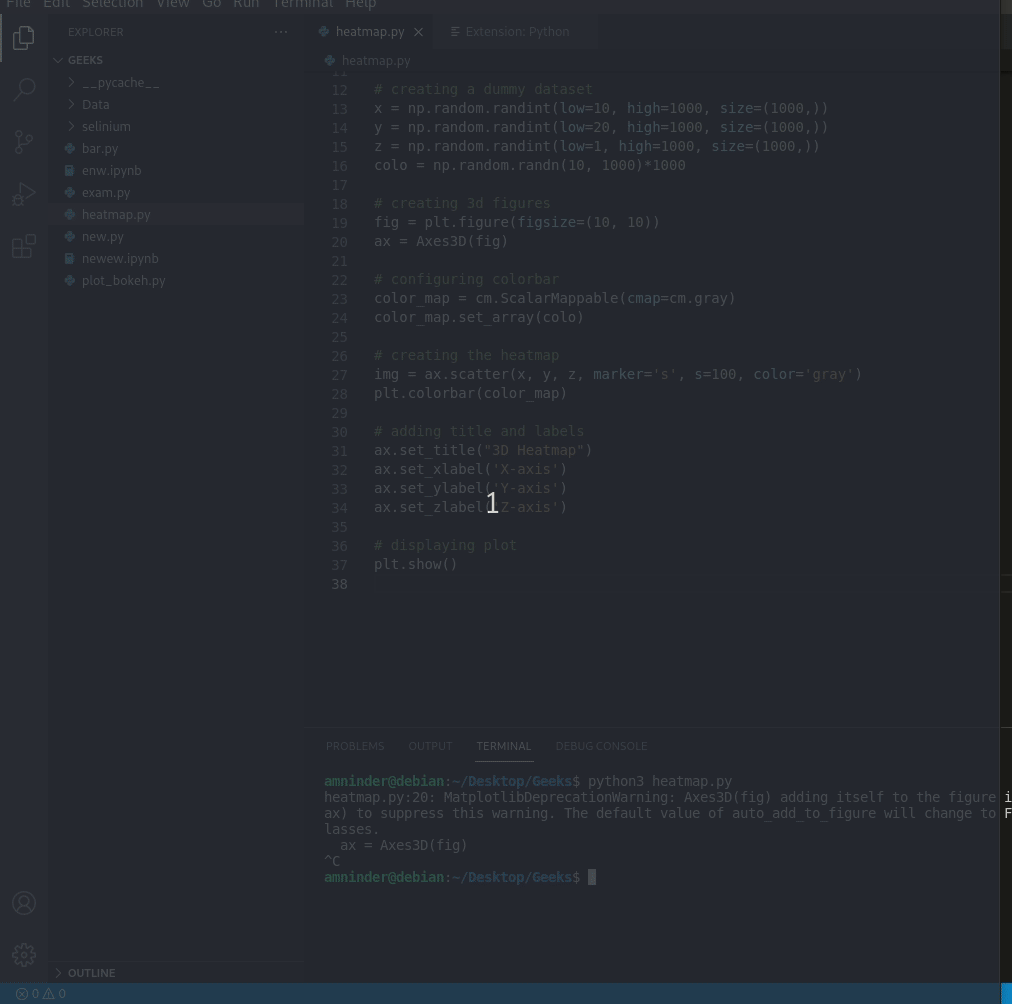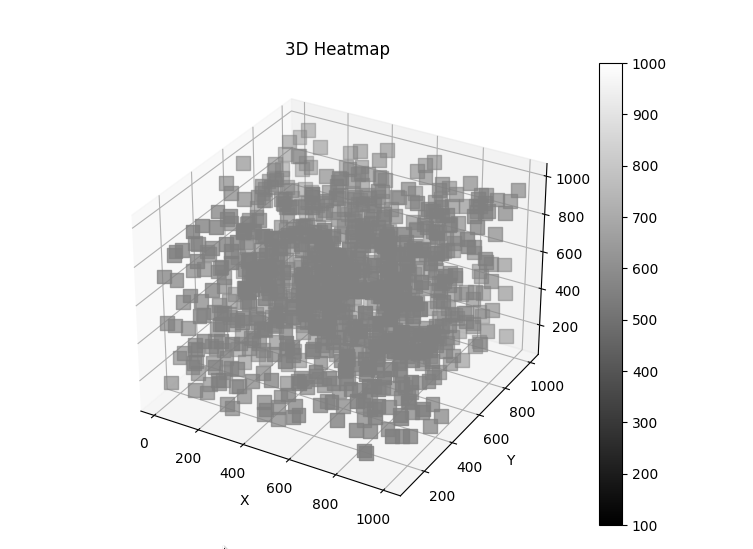# 3D Heatmap in Python

Heatmaps are a great way to visualize a dataset, methods for visualizing the data are getting explored constantly and 3D heatmap is one of the ways to plot data. Let’s learn how we can plot 3D data in python. We are going to use matplotlib and mplot3d to plot the 3D Heatmap in Python. We need to install the matplotlib explicitly by running the following command in the console:

`pip3 install matplotlib`

## Creating 3D heatmap

• In this code example, firstly we are using the statement %matplotlib to display all matplotlib plots inline within the jupyter notebook. Then we have imported all the necessary libraries needed for running this code example – the mplot3d library is used to draw 3d plots, pyplot is mainly for plotting the figure and its configuration.
• After this, we have created a random dataset for our 3d heatmap using NumPy randint function to create a random integer array.
• Using plt.figure, we have created a figure of size 10×10 width and height respectively by default the matplotlib will produce 2D plots, so to specify this as a 3d plot we use add_subplot function with projection=’3d’ to create a 3d plot.
• We are mapping an array of integers as an RGBA color for our plot using the set_array() function.
• After that, we are creating a scatter plot with our 3d dataset and by setting marker value as s we are displaying each data point as square-shaped. Their color will depend on the array that we have created earlier called colo.
• At last, we have set the x, y, z labels and title using the set_label function and displayed the plot using show() function.

Code:

## Python3

 `# 3D Heatmap in Python using matplotlib ` ` `  `# to make plot interactive  ` `%``matplotlib ` ` `  `# importing required libraries ` `from` `mpl_toolkits.mplot3d ``import` `Axes3D ` `import` `matplotlib.pyplot as plt ` `import` `numpy as np ` `from` `pylab ``import` `*` ` `  `# creating a dummy dataset ` `x ``=` `np.random.randint(low``=``100``, high``=``500``, size``=``(``1000``,)) ` `y ``=` `np.random.randint(low``=``300``, high``=``500``, size``=``(``1000``,)) ` `z ``=` `np.random.randint(low``=``200``, high``=``500``, size``=``(``1000``,)) ` `colo ``=` `[x ``+` `y ``+` `z] ` ` `  `# creating figures ` `fig ``=` `plt.figure(figsize``=``(``10``, ``10``)) ` `ax ``=` `fig.add_subplot(``111``, projection``=``'3d'``) ` ` `  `# setting color bar ` `color_map ``=` `cm.ScalarMappable(cmap``=``cm.Greens_r) ` `color_map.set_array(colo) ` ` `  `# creating the heatmap ` `img ``=` `ax.scatter(x, y, z, marker``=``'s'``, ` `                 ``s``=``200``, color``=``'green'``) ` `plt.colorbar(color_map) ` ` `  `# adding title and labels ` `ax.set_title(``"3D Heatmap"``) ` `ax.set_xlabel(``'X-axis'``) ` `ax.set_ylabel(``'Y-axis'``) ` `ax.set_zlabel(``'Z-axis'``) ` ` `  `# displaying plot ` `plt.show() `

Output:## Creating 3D heatmap with variance Dataset

• We have created a random dataset for our 3d heatmap using NumPy randint function to create a random integer array.
• Using plt.figure, we have created a figure of size 10×10 width and height respectively by default the matplotlib will produce 2D plots, so to specify this as a 3d plot we use the Axes3D to create a 3d plot. We are mapping an array of integers as an RGBA color for our plot using the set_array() function.
• After that, we are creating a scatter plot with our 3d dataset and by setting marker value as s we are displaying each data point as square-shaped. Their color will depend on the array that we have created earlier called colo.
• At last, we have set the x, y, z labels and title using the set_label function and displayed the plot using show() function.

Code :

## Python3

 `# 3D Heatmap in Python using matplotlib ` ` `  `# to make plot interactive ` `%``matplotlib inline ` ` `  `# importing required libraries ` `from` `mpl_toolkits.mplot3d ``import` `Axes3D ` `import` `matplotlib.pyplot as plt ` `import` `numpy as np ` `from` `pylab ``import` `*` ` `  `# creating a dummy dataset ` `x ``=` `np.random.randint(low``=``10``, high``=``1000``, size``=``(``1000``,)) ` `y ``=` `np.random.randint(low``=``20``, high``=``1000``, size``=``(``1000``,)) ` `z ``=` `np.random.randint(low``=``1``, high``=``1000``, size``=``(``1000``,)) ` `colo ``=` `np.random.randn(``10``, ``1000``)``*``1000` ` `  `# creating 3d figures ` `fig ``=` `plt.figure(figsize``=``(``10``, ``10``)) ` `ax ``=` `Axes3D(fig) ` ` `  `# configuring colorbar ` `color_map ``=` `cm.ScalarMappable(cmap``=``cm.gray) ` `color_map.set_array(colo) ` ` `  `# creating the heatmap ` `img ``=` `ax.scatter(x, y, z, marker``=``'s'``, ` `                 ``s``=``100``, color``=``'gray'``) ` `plt.colorbar(color_map) ` ` `  `# adding title and labels ` `ax.set_title(``"3D Heatmap"``) ` `ax.set_xlabel(``'X-axis'``) ` `ax.set_ylabel(``'Y-axis'``) ` `ax.set_zlabel(``'Z-axis'``) ` ` `  `# displaying plot ` `plt.show() `

Output:## Creating 3D heatmap with CSV file

• We are using pandas we have loaded the dataset and we are using 3 columns for plotting and one column for color bar. You can operate the data as your wish.
• Using plt.figure, we have created a figure of size 8×5 width and height respectively by default the matplotlib will produce 2D plots, so to specify this as a 3d plot we use add_subplot function with projection=’3d’ to create a 3d plot. We are mapping an array of integers as an RGBA color for our plot using the set_array() function.
• After that, we are creating a scatter plot with our 3d dataset and by setting marker value as s we are displaying each data point as square-shaped. Their color will depend on the array that we have created earlier called colo.
• At last, we have set the x, y, z labels and title using the set_label function and displayed the plot using show() function.

 `#!/usr/bin/python3 ` `# 3D Heatmap in Python using matplotlib ` ` `  `# to make plot interactive ` `%``matplotlib inline ` ` `  `# importing required libraries ` `from` `mpl_toolkits.mplot3d ``import` `Axes3D ` `import` `matplotlib.pyplot as plt ` `import` `numpy as np ` `import` `pandas as pd ` `from` `pylab ``import` `*` ` `  `# reading a dummy dataset ` `dataset ``=` `pd.read_csv(``"/data.csv"``) ` `x ``=` `dataset[``"Col.1"``].tolist() ` `y ``=` `dataset[``"Col.2"``].tolist() ` `z ``=` `dataset[``"Col.3"``].tolist() ` ` `  `colo ``=` `dataset[``"total"``].tolist() ` ` `  `# creating 3d figures ` `fig ``=` `plt.figure(figsize``=``(``8``, ``5``)) ` `ax ``=` `fig.add_subplot(``111``, projection``=``'3d'``) ` ` `  `# configuring colorbar ` `color_map ``=` `cm.ScalarMappable(cmap``=``cm.gray) ` `color_map.set_array(colo) ` ` `  `# creating the heatmap ` `img ``=` `ax.scatter(x, y, z, marker``=``'s'``,  ` `                 ``s``=``99``, color``=``'gray'``) ` `plt.colorbar(color_map) ` ` `  `# adding title and labels ` `ax.set_title(``"3D Heatmap"``) ` `ax.set_xlabel(``'X'``) ` `ax.set_ylabel(``'Y'``) ` `ax.set_zlabel('') ` ` `  `# displaying plot ` `plt.show() `